NCERT Solutions for Class 6 Maths Chapter 11 Algebra Ex 11.1 are part of NCERT Solutions for Class 6 Maths. Here we have given NCERT Solutions for Class 6 Maths Chapter 11 Algebra Ex 11.1.

 Board CBSE Textbook NCERT Class Class 6 Subject Maths Chapter Chapter 11 Chapter Name Algebra Exercise Ex 11.1 Number of Questions Solved 1 Category NCERT Solutions

## NCERT Solutions for Class 6 Maths Chapter 11 Algebra Ex 11.1

Question 1.
Find the rule which gives the number of matchsticks required to make the following matchstick patterns. Use a variable to write the rule.
(a) A pattern of letter T as(b) A pattern of letter Z as(c) A pattern of letter U as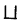(d) A pattern of letter V as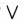(e) A pattern of letter E as(f) A pattern of letter S as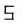(g) A pattern of letter A asSolution.
(a) Number of matchsticks required = 2n
(b) Number of matchsticks required = 3n
(c) Number of matchsticks required = 3n
(d) Number of matchsticks required = 2n
(e) Number of matchsticks required = 5n
(f) Number of matchsticks required = 5n
(g) Number of matchsticks required = 6

Question 2.
We already know the rule for the pattern of letters L, C and F. Some of the letters from Q. 1 (given above) give us the same rule as that given by L. Which are these? Why does this happen?
Solution.
These letters are T and V. This happens since the number>of matchsticks require,d in each of them is 2.

Question 3.
Cadets are marching in a parade. There are 5 cadets in a row. What is the rule, which gives the number of cadets, given the number of rows? (Use n for the number of rows.)
Solution.
The number of cadets = 5n.

Question 4.
If there are 50 mangoes in a box, how will you write the total number of mangoes in terms of the number of boxes? (Use b for the number of boxes.)
Solution.
Total number of mangoes = 50b.

Question 5.
The teacher distributes 5 pencils per student. Can you tell how many pencils are needed, given the number of students? (Use for the number of students.)
Solution.
Number of pencils needed = 5s

Question 6.
A bird flies 1 kilometer in one minute. Can you express the distance covered by the bird in tertns of its flying time in minutes? (Use t for flying time in minutes.)
Solution.
Yes! / kilometers
The bird flies in one minute = 1 kilometer
The bird flies in / minutes = 1 x t kilometers
= kilometers

Question 7.
Radha is drawing a dot Rangoli (a beautified pattern of lines joining dots with chalk powder. She has 9 dots in a row. How many dots will her Rangoli have for r rows? How many dots are there if there are 8 rows? If there are 10 rows?Solution.
∵ Number of dots in 1 row = 9
∴ Number of dots in r rows = 9 x r=9r
Number of dots in 8 rows = 9 x 8 = 72
Number of dots in 10 row = 9 x 10 = 90

Question 8.
Leela is Radha’s younger sister. Leela is 4 years younger than Radha. Can you write Leela’s age in terms of Radha’s age? Take Radha’s age to be x years.
Solution.
Yes! we can write Leela’s age in terms of Radha’s age.
Age of Radha = x years
∵ Leela is 4 years younger than Radha.
∴ Age of Leela = (x – 4) years

Question 9.
Solution.
Number of laddus given away to guests and family members = l
Number of laddus remained = 5

Question 10.
Oranges are to be transferred from larger boxes into smaller boxes. When a large box is emptied, the oranges from it fill two smaller boxes and still, 10 oranges remain, outside. If the number of oranges in a small box is taken to be x, what is the number of oranges in the larger box?
Solution.
Let the number of oranges in a smaller box box
∴ Number of oranges in two smaller boxes = 2x
Number of oranges remained outside = 10
∴ Number of oranges in the larger box = 2x+ 10

Question 11.
(a) Look at the following matchstick pattern of squares (figure). The squares are not separate. Two neighboring squares have a common matchstick. Observe the pattern and find the rule that gives the number of matchsticks in terms of the number of squares.
(Hint: If you remove the vertical stick at the end, you will get a pattern of Cs.)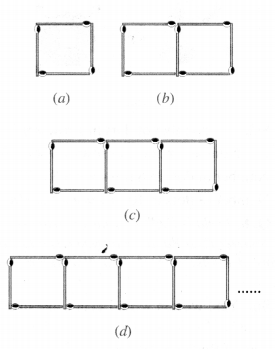(b) Figure gives a matchstick pattern of triangles. Av in Exercise 11(a) above, find the general rule that gives the number of matchsticks in terms of the number of triangles.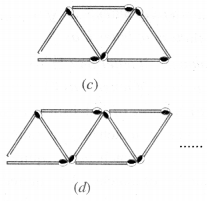Solution.
(a)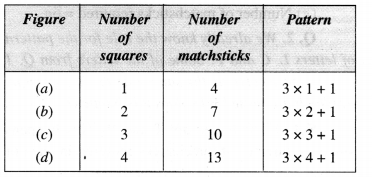Rule: Number of matchsticks required = 3x + I
where x is the number of squares.

(b)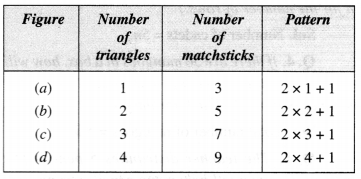Rule: Number of matchsticks required = 2x + 1,
where x is the number of triangles.

We hope the NCERT Solutions for Class 6 Maths Chapter 11 Algebra Ex 11.1 help you. If you have any query regarding. NCERT Solutions for Class 6 Maths Chapter 11 Algebra Ex 11.1, drop a comment below and we will get back to you at the earliest.